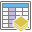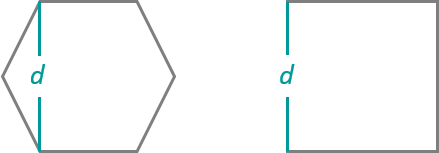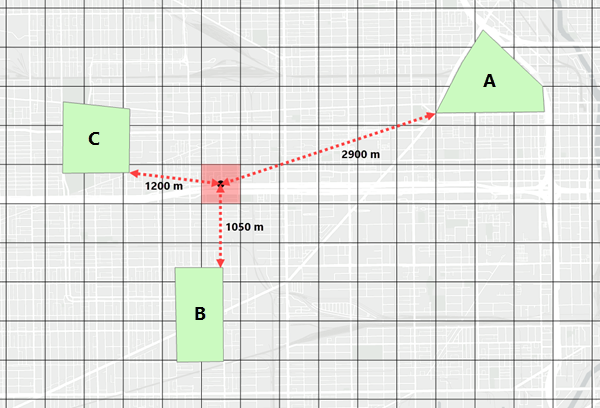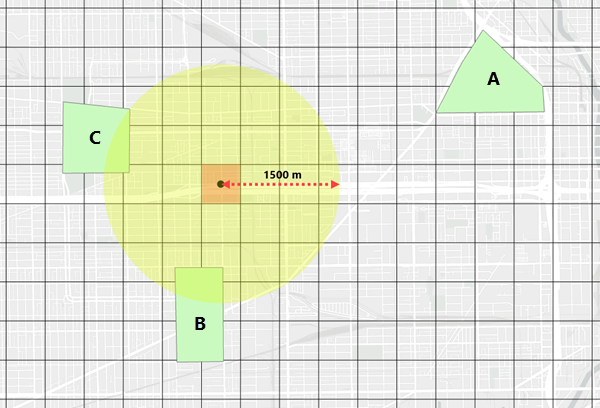Build Multi-Variable GridThe Build Multi-Variable Grid tool generates a grid of square or hexagonal bins and calculates variables for each bin based on the proximity of one or more input layers. These variables can include the following:

• Distance to Nearest—The distance from each bin to the nearest feature
• Attribute of Nearest—A field value of the feature nearest to each bin
• Summary of Nearby—A specified statistic of all features within a distance of each bin
• Summary of Intersecting—A specified statistic of all features that intersect each bin

Analysis using GeoAnalytics Tools

Analysis using GeoAnalytics Tools is run using distributed processing across multiple ArcGIS GeoAnalytics Server machines and cores. GeoAnalytics Tools and standard feature analysis tools in ArcGIS Enterprise have different parameters and capabilities. To learn more about these differences, see Feature analysis tool differences.

Examples

Choosing where to invest in new public transportation infrastructure can be a complicated problem requiring the visualization of many types of data. You have access to multiple datasets representing existing infrastructure throughout the United States, as well as several layers of census data. You can use Build Multi-Variable Grid to summarize all of this data into a single dataset, with which you can visualize the distance to the nearest existing infrastructure, the mean surrounding population, and the variance of surrounding income for every location in the United States.

Usage notes

Variables can be calculated for point, line, or area features.

For each variable, you must specify the variable type and provide a title. This title will be the name of the field in the result layer that contains the values for each bin. Each variable type that can be calculated using Build Multi-Variable Grid must be configured with a unique set of parameters. These parameters are listed below.

VariableDescriptionParameter

Distance to Nearest

The distance from the center of each bin to the nearest feature in the input layer. The result will be in the units of the processing spatial reference (see setting the processing spatial reference).

Maximum distance from bin center is the farthest from the center of each bin that the tool will search for a feature in the input layer. This value must be larger than the bin size. If no feature is found within this search radius, the bin will be assigned a value of null for this variable.

Attribute of Nearest

A field value of the feature nearest to each bin center. The field can be of any type. If two or more features are equidistant from the bin center and also the closest, one of the features will be chosen at random and its field value will be included in the result layer.

Field to include is the name of a field in the input layer. The value of this field in the feature closest to the center of a bin will be included as an attribute of the bin in the result layer.

Maximum distance from bin center is the farthest distance from the center of each bin that the tool will search for a feature in the input layer. This value must be larger than the bin size. If no feature is found within this search radius, the bin will be assigned a value of null for this variable.

Summary of Nearby

A statistic calculated on a specified field and using all features located within a given distance of each bin center. A feature will be included in calculations if any portion of it lies within this distance.

Statistic to calculate is the name of a field in the input layer and a statistic to calculate on that field.

Summarize features within is the farthest distance from the center of each bin that the tool will search for features in the input layer. All features found within this search radius will be summarized using the specified statistic. This value must be larger than the bin size. If no features are found within this search radius, the bin will be assigned a value of null for this variable.

Summary of Intersecting

A statistic calculated on a specified field and using all features that intersect a bin. If any portion of a feature lies within the area of a bin, the feature will be included in calculations.

Statistic to calculate is the name of a field in the input layer and a statistic to calculate on that field. For each bin, features that intersect the bin will be summarized. If no features intersect the bin, it will be assigned a value of null for this variable.

For the Summary of Nearby and Summary of Intersecting options, you can calculate the following statistics on numeric fields:

• Count—Calculates the number of nonnull values. It can be used on numeric fields or strings. The count of [null, 0, 2] is 2.
• Sum—The sum of numeric values in a field. The sum of [null, null, 3] is 3.
• Mean—The mean of numeric values. The mean of [0, 2, null] is 1.
• Min—The minimum value of a numeric field. The minimum of [0, 2, null] is 0.
• Max—The maximum value of a numeric field. The maximum value of [0, 2, null] is 2.
• Range—The range of a numeric field. This is calculated as the minimum values subtracted from the maximum value. The range of [0, null, 1] is 1. The range of [null, 4] is 0.
• Variance—The variance of a numeric field in a track. The variance of  is null. The variance of [null, 1,0,1,1] is 0.25.
• Standard deviation—The standard deviation of a numeric field. The standard deviation of  is null. The standard deviation of [null, 1,0,1,1] is 0.5.
On string fields, you can calculate the following:
• Count—The number of nonnull strings.
• Any—This statistic is a random sample of a string value in the specified field.
All statistics are calculated on nonnull values.

Bin size specifies how large the bins of your multivariable grid are. If you choose to generate hexagonal bins, the size is the height of each hexagon, and the width of the resulting hexagon will be 2 times the height divided by the square root of 3. If you choose to generate square bins, the bin size is the height of the square, which is equal to the width.GeoAnalytics Tools analysis using binning (hexagon or square) with a specified geographic coordinate system will automatically use a projected coordinate system based on the extent of the data. To learn more about setting your coordinate system for analysis, see Use the analysis environments for GeoAnalytics Tools in Map Viewer.

If Use current map extent is checked, only the features that are visible within the current map extent will be analyzed. If it's not checked, all input features in the input layer will be analyzed, even if they are outside the current map extent.

Limitations

Build Multi-Variable Grid does not work with tables. Input layers must be points, lines, or areas.

How Build Multi-Variable Grid works

Distance calculations

All distances in the Build Multi-Variable Grid tool are measured from the center of bins. For both square and hexagonal bins, the center is located at half the bin height and half the bin width, as shown below.For Distance to Nearest and Attribute of Nearest, the distance to polygon or line features is measured using the closest point in the feature to the bin of interest. Distances to point features are measured using the point location.Summary calculations

For Summary of Nearby, features are included in summary statistic calculations if any part falls within a circle surrounding the bin center with a radius determined by the Summarize features within parameter. For Summary of Intersecting, features are included in calculations if any part falls within the boundaries of a bin area (no circular radius is used).Understanding results

Any bin with all null attributes will not be included in the result. This means that the result layer will span the maximum extent of your input features plus the largest search distance of the variables specified (within the extent of the processing spatial reference).

Each variable that you configure will become a field in the attribute table of the result layer. No fields from the input layers will be included in the result automatically; you must specify the information to include by configuring variables.

ArcGIS API for Python example

The Build Multi-Variable Grid tool is available through ArcGIS API for Python.

This example creates a multivariable grid by summarizing information such as the distance to nearest road or intersection, the mean age of the surrounding population, and the variance of income in the surrounding population.

# Import the required ArcGIS API for Python modules
import arcgis
from arcgis.gis import GIS
from arcgis.geoanalytics import summarize_data
from arcgis.features import FeatureLayer

# Connect to your ArcGIS Enterprise portal and check that GeoAnalytics is supported
portal = GIS("https://myportal.domain.com/portal", "gis_publisher", "my_password", verify_cert=False)
if not portal.geoanalytics.is_supported():
print("Quitting, GeoAnalytics is not supported")
exit(1)

# Find the big data file share dataset you're interested in using for analysis
search_result = portal.content.search("", "Big Data File Share")

# Look through search results for a big data file share with the matching name
bd_file = next(x for x in search_result if x.title == "bigDataFileShares_CityData")

# Look through the big data file share for roads

# Look through the big data file share for intersections
intersections = next(x for x in bd_file.layers if x.properties.name == "intersections")

# Find a feature layer named "Demographics" in your ArcGIS Enterprise portal
demographics = portal.content.search("Demographics", "Feature Layer")
demographics_layer = layer_result.layers

variables = [
{
"layer":0,
"variables":[
{
"type":"DistanceToNearest",
"searchDistance":20,
"searchDistanceUnit":"Kilometers",
"filter":"Rural = 'false'"
}
]
},
{
"layer":1,
"variables":[
{
"type":"AttributeOfNearest",
"outFieldName":"intersection",
"attributeField":"intersection_name",
"searchDistance":50,
"searchDistanceUnit":"Kilometers"
}
]
},
{
"layer":2,
"variables":[
{
"type":"AttributeSummaryOfRelated,
"outFieldName":"MeanPopAge",
"statisticType":"Mean",
"statisticField":"Age",
"searchDistance":50,
"searchDistanceUnit":"Kilometers"
},
{
"type":"AttributeSummaryOfRelated,
"outFieldName":"VarIncome",
"statisticType":"Variance",
"statisticField":"Income",
"searchDistance":50,
"searchDistanceUnit":"Kilometers"

}
]
}
]

# Set the tool environments
arcgis.env.verbose = True
arcgis.env.defaultAggregations = True

# Run the Build Multi-Variable Grid tool
output = summarize_data.build_multivariable_grid(input_layers = inputs,
variable_calculations = variables,
bin_size = 10,
bin_unit = "Kilometers",
bin_type = "Hexagon",
output_name = "CityPlanningGrid")

# Visualize the tool results if you are running Python in a Jupyter Notebook
processed_map = portal.map('City, State', 10)
processed_map

Similar tools

Use Build Multi-Variable Grid to summarize multiple layers into a single grid of polygons, either for visualization or for later use in training prediction or classification models. Other tools may be useful in solving similar but slightly different problems.

Map Viewer analysis tools

If you only want to summarize a single input layer into bins, or if you want to summarize into a polygon layer, use the Summarize Within GeoAnalytics Tools.

If you want to aggregate a single point layer into areas, use the Aggregate Points GeoAnalytics Tools.

If you are trying to summarize points, lines, or areas using different spatial relationships, use the Join Features GeoAnalytics Tools.

If you are trying to summarize lines or areas using the standard analysis tools, see Summarize Within.

If you want to aggregate points into areas using the standard analysis tools, see Aggregate Points.

ArcGIS Desktop analysis tools

Build Multi-Variable Grid performs functions similar to the Summarize Nearby and Near tools.